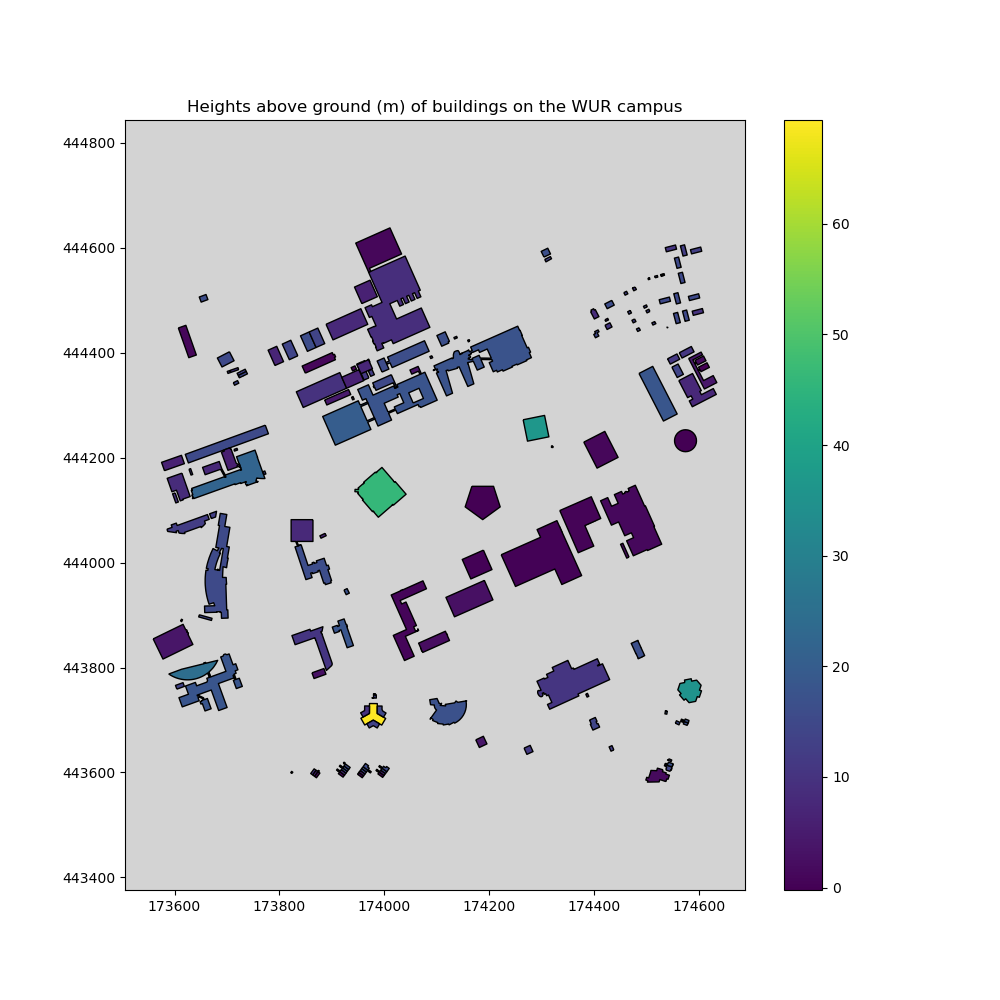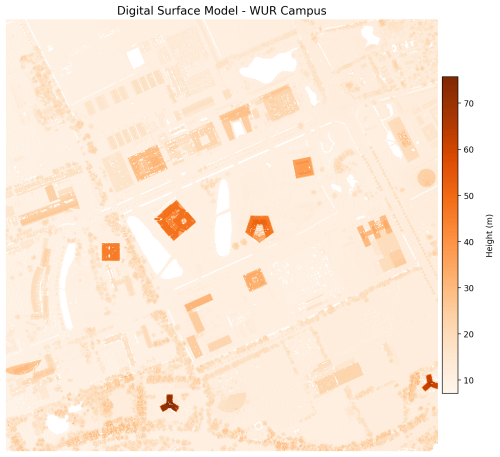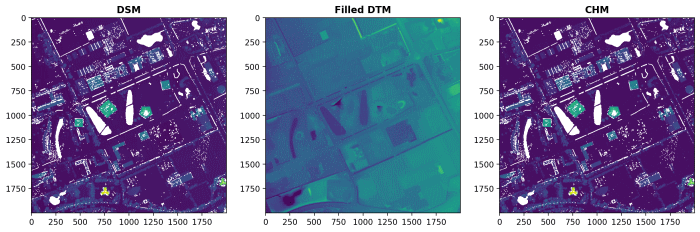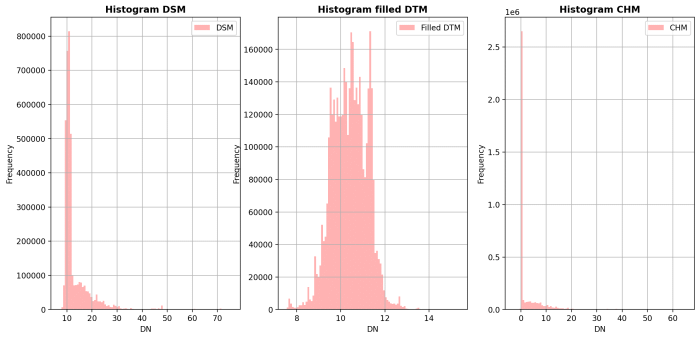Week 3, Lesson 10: Handling Raster data with Python

Jan Verbesselt, Jorge Mendes de Jesus, Aldo Bergsma, Dainius Masiliūnas, David Swinkels, Judith Verstegen, Corné Vreugdenhil - 2022-01-09

Introduction

Today we will work with Python packages for spatial raster analysis. Python has some dedicated packages to handle rasters:

• GDAL is powerful library for reading, writing and warping raster datasets
• Rasterio reads and writes geospatial raster data
• NumPy is fundamental package for scientific computing, such as array (thus raster) calculations
• rasterstats summarizes geospatial raster datasets based on vector geometires

Today’s Learning objectives

• Be able to read spatial raster formats from web services and files
• Be able to write spatial raster formats to disk
• Know how to apply basic operations on raster data, such as arithmetics
• Be able to plot spatial raster data with matplotlib

Setting up the Python Environment

Make a folder structure for this tutorial:

cd ~/Documents/
mkdir PythonRaster #or give the directory a name to your liking
cd ./PythonRaster
mkdir data
mkdir output

Next, create a text file, (re)name it (to) raster.yaml, and copy the following content into the file:

name: raster
channels:
- conda-forge
dependencies:
- python
- numpy
- matplotlib
- spyder
- owslib
- gdal
- rasterio
- geopandas
- rasterstats
- affine

Use this file to create an environment for this tutorial, as you have learned in the refresher lesson. NOTE: This can take a while (5-10 minutes); just go and get a coffee/tea/water/juice. Activate the environment, open Spyder, create a script in the root folder and start coding.

Via a Web Coverage Service

A Web Coverage Service (WCS) loads raster data in a similar way as Web Feature Services (WFS) load vector data. Web Coverage Services are a standard by the Open Geospatial Consortium and allow the downloading of geospatial raster data with multiple types of format encoding: GeoTIFF, netCDF, JPEG2000 etc. A Web Map Service [WMS] also exists for rasters; it allows downloading of images but without the data values.

Today we will work with elevation rasters. Have a look at the WCS of the AHN dataset. AHN stands for “Actueel Hoogtebestand Nederland” and is a Digital Elevation Model [DEM] that covers the Netherlands. Access the web coverage service to have a look at the contents.

from owslib.wcs import WebCoverageService

# Access the WCS by proving the url and optional arguments (here version)
wcs = WebCoverageService('http://geodata.nationaalgeoregister.nl/ahn2/wcs?service=WCS', version='1.0.0')
# Print to check the contents of the WCS
print(list(wcs.contents))

Running the last line of code shows that the Web Coverage Service of the AHN2 contains four rasters: 0.5m interpolated, 0.5m not interpolated, 0.5m rough, and 5m. The meters identify the cell size. Raster data of AHN has the projected coordinate system RD_New (EPSG: 28992).

We can also check what types of operations are available in the WCS:

# Get all operations and print the name of each of them
print([op.name for op in wcs.operations])

You will see that the Web Coverage Service allows accessing the data (GetCoverage), the metadata (DescribeCoverage), and the capabilities (GetCapabilities). These are all standard protocols defined by the OGC.

Several functions are available to access specific metadata of each individual raster, for example:

# Take the 0.5m-resolution rough raster as example
cvg = wcs.contents['ahn2_05m_ruw']
# print supported reference systems, the bounding box defined in WGS 84 coordinates, and supported file formats
print(cvg.supportedCRS)
print(cvg.boundingBoxWGS84)
print(cvg.supportedFormats)

Question 1: What is the coordinate reference system of the 0.5m rough raster? Is it the same for the other three rasters?

Let us have a look at the data. We do not want to overload the web service. Therefore, we download once and store the data locally.

Download the Digital Surface Model [DSM], which is the the ‘ahn2_05m_ruw’ version, and Digital Terrain Model [DTM], which is the ‘ahn2_05m_int’ version, to a local file. The difference between a DEM, DSM and DTM is explained on the GIS StackExchange.

import os

# Define a bounding box in the availble crs (see before) by picking a point and drawing a 1x1 km box around it
x, y = 174100, 444100
bbox = (x-500, y-500, x+500, y+500)

# Create a data directoty within the directory where this script is run if it does not exist yet and store file
if not os.path.exists('data'): os.makedirs('data')

# Request the DSM data from the WCS
response = wcs.getCoverage(identifier='ahn2_05m_ruw', bbox=bbox, format='GEOTIFF_FLOAT32', crs='urn:ogc:def:crs:EPSG::28992', resx=0.5, resy=0.5)

# Write the data to a local file in the 'data' folder (it should exist)
with open('./data/AHN2_05m_DSM.tif', 'wb') as file:

# Do the same for the DTM
response = wcs.getCoverage(identifier='ahn2_05m_int', bbox=bbox, format='GEOTIFF_FLOAT32', crs='urn:ogc:def:crs:EPSG::28992', resx=0.5, resy=0.5)

with open('./data/AHN2_05m_DTM.tif', 'wb') as file:

From a file with GDAL

GDAL handles raster and vector geospatial data formats with Python, Java, R and C APIs. When opening a raster file in gdal, the object has a hierarchical structure starting at the Dataset level. A Dataset has a Geotransform (metadata) and can contain one or more Bands. Each Band has a Data array and potentially Overviews.

Let us open the file we just saved. You will see you first get the dataset, and need to access the band (even though there is only one), before the data array can be accessed.

from osgeo import gdal

# Open dataset, gdal automatically selects the correct driver
ds = gdal.Open("./data/AHN2_05m_DTM.tif" )

# Get the band (band number 1)
band = ds.GetRasterBand(1)

# Get the data array
print(data)

# Delete objects to close the file
ds = None

Question 2: Why do we set ds to None at the end of your script? What may happen if you do not do that?

The GDAL Python API is not the best documented Python module. Therefore, Rasterio is explained as an alternative raster data handling module.

From a file with Rasterio

Rasterio reads and writes multiple raster formats based on GDAL, provides raster processing functions based on NumPy arrays and GeoJSON, and integrates matplotlib in the module rasterio.plot for visualization. Rasterio handles multiple bands, masking, reprojecting, and resampling.

The rest of the lesson below is a complete route of handling a rasterdataset. We will use the DEMs from a the WCS for our study area, handle it with Rasterio, calculate new information (CHM), overlay it with vector data representing buildings and visualize it.

Let us read in the raster data we just stored from the WCS with rasterio and plot it with rasterio.plot:

import rasterio
from rasterio.plot import show

# open the two rasters
dsm = rasterio.open("./data/AHN2_05m_DSM.tif", driver="GTiff")
dtm = rasterio.open("./data/AHN2_05m_DTM.tif", driver="GTiff")

print(dsm.meta)
print(dtm.meta)

# plot with rasterio.plot, which provides matplotlib functionality
show(dsm, title='Digital Surface Model', cmap='gist_ncar')Question 3: Is the colormap ‘gist_ncar’ cartographically a good choice for a digital surface model? Why/Why not? If not, try to find a more fitting one.

The metadata shows the driver (GDAL’s way of knowing how to function with a specific file format), datatype, nodata value, width of raster in number of cells, height of raster in number of cells, number of raster bands in the dataset, coordinate reference system, and transformation values.

In the back-end, raster layers in rasterio are stored as NumPy arrays, which appears when the data are read with the method .read():

# rasterio object
print(type(dsm))

# read, show object type and data
print(type(dsm_data))
print(dsm_data)

Processing raster data

Computing a Canopy Height Model

A Canopy Height Model (CHM) gives an indication of the height of trees. It can be created by subtracting a Digital Terrain Model from a Digital Surface Model. In the resulting raster, each cell value represents the tree overstory height above the underlying surface topography. Rasterio relies on NumPy to perform raster point operations.

# access the data from the two rasters
# subtract the NumPy arrays
chm = dsm_data - dtm_data
# check the resulting array
print(chm)

# Copy metadata of one of the rasters (does not matter which one)
kwargs = dsm.meta
# save the chm as a raster
with rasterio.open('./data/AHN2_05m_CHM.tif', 'w', **kwargs) as file:
file.write(chm.astype(rasterio.float32))

Question 4: Where is the canopy the highest in the study area? Is that what you expected?

Although we have applied the concepts of a Canopy Height Model, the area we picked does not only contain vegetation on top of the surface, but also buildings. The heights of vegetation and buildings are thus currently combined in our chm raster.

Computing heights of buildings

Let us determine the heights of buildings only. First step is to download building data from the BAG Web Feature Service that we used in the vector tutorial.

import geopandas as gpd
from requests import Request

# bounding box (same as in previous script)
x, y = 174100, 444100
bbox = (x-500, y-500, x+500, y+500)

# extract only buildings on and around WUR campus
url = 'https://geodata.nationaalgeoregister.nl/bag/wfs/v1_1'
layer = 'bag:pand' # see wfs.contents
bb = ','.join(map(str, bbox)) # string of bbox needed for the request url

# Specify the parameters for fetching the data
params = dict(service='WFS', version="1.1.0", request='GetFeature',
typeName=layer, outputFormat='json',
srsname='urn:ogc:def:crs:EPSG::28992', bbox=bb)

# Parse the URL with parameters
q = Request('GET', url, params=params).prepare().url

Question 5: What happens in the line “bb = ‘,’.join(map(str, bbox))”? Look up how mapping works if you do not know.

Next step is to perform zonal statistics to get the average height value per building polygon. We will do this with the module Rasterstats, which uses GeoDataFrames and .tif files for this task. It outputs a GeoJSON.

import rasterstats as rs

# apply the zonal statistics function with gdf and tif as input
chm_buildings = rs.zonal_stats(buildings_gdf, "./data/AHN2_05m_CHM.tif", prefix='CHM_', geojson_out=True)
# convert geojson to GeoDataFrame
buildings_gdf = gpd.GeoDataFrame.from_features(chm_buildings)
# check the added attributes with a prefix 'CHM_'
print(buildings_gdf['CHM_mean'])

You may get a warning message from rasterstats; you can ignore this.

A quick visualization shows us the heights derived from the raster data on the map:

import matplotlib.pyplot as plt

# Create one plot with figure size 10 by 10
fig, ax = plt.subplots(1, figsize=(10, 10))

# Customize figure with title, legend, and facecolor
ax.set_title('Heights above ground (m) of buildings on the WUR campus')
buildings_gdf.plot(ax=ax, column='CHM_mean', k=6,
cmap=plt.cm.viridis, linewidth=1, edgecolor='black', legend=True)
ax.set_facecolor("lightgray")

# Make sure to get an equal scale in the x and y direction
plt.axis('equal')

# Visualize figure
plt.show()Question 6: Why do we want an equal scale in the x and y direction for this figure?

Writing raster data to a file

To store the NumPy array as a raster file, Rasterio needs the accompanying metadata, as you’ve seen before. It is possible to use the metadata of an existing raster, or to create it from scratch.

To create metadata from scratch, the CRS can be defined with a function from Rasterio and the transformation with Affine. Affine is a Python module that facilitates affine transformations, i.e. scaling, rotating, mirroring or skewing of images/rasters/arrays.

Rasterio can write most raster formats from GDAL. The developers recommend using GeoTiff driver for writing as it is the best-tested and best-supported format.

import affine

# specify the components of the crs (we know them from the DSM)
kwargs = {'driver': 'GTiff',
'dtype': 'float32',
'nodata': None,
'width': 2000,
'height': 2000,
'count': 1,
'crs': rasterio.crs.CRS({'init': 'epsg:28992'}),
'transform': affine.Affine(0.5, 0.0, 173600.0, 0.0, -0.5, 444600.0)}

# write the raster file (notice that it is the old chm; chm_buildings is not a raster)
with rasterio.open('./data/AHN2_05m_CHM.tif', 'w', **kwargs) as file:
file.write(chm.astype(rasterio.float32))

Visualizing raster data

Raster data can be visualized by passing NumPy arrays to Matplotlib directly or secondly via a method in Rasterio that accesses Matplotlib for you. Using Matplotlib directly allows more flexibility, such as tweaking the legend, axis and labels, and is more suitable for professional purposes. The visualization via Rasterio requires less code and can give a quick idea of your raster data. We will show both approaches. Let us make a visualization of the DSM and DTM with Matplotlib:

import matplotlib.pyplot as plt

# Create one plot with figure size 10 by 10
fig, ax = plt.subplots(figsize=(10,10))

# Imshow is the main raster plotting method in matplotlib
# Again, ensure an equal scale in the x and y direction
dsmplot = ax.imshow(dsm.read(1), cmap='Oranges', extent=bbox, aspect='equal')

# Title (do not do this for a scientific report, use a caption instead)
ax.set_title("Digital Surface Model - WUR Campus", fontsize=14)

# Legend with label
cbar = fig.colorbar(dsmplot, fraction=0.035, pad=0.01, extend='both')
cbar.ax.set_ylabel('Height (m)', rotation=90)

# Hide the axes
ax.set_axis_off()
plt.show()

# Same procedures for the DTM
fig, ax = plt.subplots(figsize=(10,10))
dtmplot = ax.imshow(dtm.read(1), cmap='Oranges', extent=bbox, aspect='equal')
ax.set_title("Digital Terrain Model - WUR Campus", fontsize=14)
cbar = fig.colorbar(dtmplot, fraction=0.035, pad=0.01, extend='both')
cbar.ax.set_ylabel('Height (m)', rotation=90)
ax.set_axis_off()
plt.show()If you do not like the orange colormap of Matplotlib, it is possible to pick another colormap.

The second approach with Rasterio only requires one line of code to make a plot. By creating subplots, the figures can be combined (can be done with Matplotlib directly as well).

from rasterio.plot import show

# Figure with three subplots, upack directly
fig, (axdsm, axdtm, axchm) = plt.subplots(1, 3, figsize=(21, 7))

# Populate the three subplots with raster data
show(dsm, ax=axdsm, title='DSM')
show(dtm, ax=axdtm, title='DTM')
show(chm, ax=axchm, title='CHM')
plt.show()Rasterio also visualizes simple histograms by calling functions of Matplotlib.

from rasterio.plot import show_hist

# Figure with three subplots, upack directly
fig, (axdsm, axdtm, axchm) = plt.subplots(1, 3, figsize=(21, 7))

# Populate the three subplots with histograms
show_hist(dsm, ax=axdsm, bins=100, lw=0.0, stacked=False, alpha=0.3, title="Histogram DSM")
show_hist(dtm, ax=axdtm, bins=100, lw=0.0, stacked=False, alpha=0.3, title="Histogram DTM")
show_hist(chm, ax=axchm, bins=100, lw=0.0, stacked=False, alpha=0.3, title="Histogram CHM")

# Build legends and show
axdsm.legend(['DSM'])
axdtm.legend(['DTM'])
axchm.legend(['CHM'])
plt.show()Question 7: What is represented on the x and y axis? The default axis labels are DN (x) and Frequency (y); if you were to change them, what labels would you pick to better reflect the content of the plots?